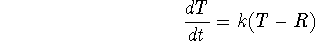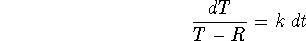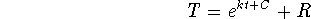Next: Example Up: Applications of the Natural Previous: Example

## Newton's Law of Cooling

Newton's Law of Cooling is used to model the temperature change of an object of some temperature placed in an environment of a different temperature. The law states thatwhere T is the temperature of the object at time t, R is the temperature of the surrounding environment (constant) and k is a constant of proportionality. What this law says is that the rate of change of temperature is proportional to the difference between the temperature of the object and that of the surrounding environment.

In order to get the previous equation to something that we can use, we must solve the differential equation. The steps are given below.

1. Separate the variables. Get all the T's on one side and all the t's on the other side. The constants can be on either side.2. Anti-differentiate both sides.3. Leave in the previous form or solve for T.We now have a useful equation. When you are working with Newton's Law of Cooling, remember that t is the variable. The other letters, R, k, C, are all constants. In order to find the temperature of the object at a given time, all of the constants must first have numerical values.

Roxanne Tisch
Tue Sep 24 13:59:38 EDT 1996